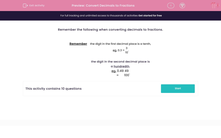# Convert Decimals to Fractions

In this worksheet, students convert decimals (to 2 decimal places) to fractions.Key stage:  KS 2

Curriculum topic:   Maths and Numerical Reasoning

Curriculum subtopic:   Decimals

Difficulty level:#### Worksheet Overview

Remember the following when converting decimals to fractions.

Remember the digit in the first decimal place is a tenth,
eg. 0.3 =
 3 10
.

the digit in the second decimal place is a hundredth.

eg. 0.49 =
 49 100
.

### What is EdPlace?

We're your National Curriculum aligned online education content provider helping each child succeed in English, maths and science from year 1 to GCSE. With an EdPlace account you’ll be able to track and measure progress, helping each child achieve their best. We build confidence and attainment by personalising each child’s learning at a level that suits them.

Get started